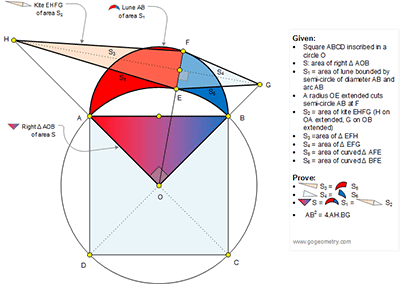## Sunday, May 7, 2017

### Geometry Problem 1335: the Lune of Hippocrates has the same area of a Kite

Geometry Problem. Post your solution in the comment box below.
Level: Mathematics Education, High School, Honors Geometry, College.

This entry contributed by Markus Heisss, Wurzburg, Bavaria.

Details: Click on the figure below..1.Problem 1335
4 .Suppose that HF intersects the circle (K,KA ) at the points P and Q (P is left of Q), OK is perpendicular to AB (K on AB) , KT perpendicular to OA (T on OA ) , KN perpendicular to PQ (N on PQ ) and KL perpendicular to OF (L on OF). Let HG intersect EF in M then
EM=MF=x and OA=R , KE=KO=KB=KA=R√(2 )/2. Is KT=KN=R/2, LF=LO=OF/2=(R/2)+x=
LM+x or LM=KN=R/2=KT. So PQ=AO=R.Therefore the point K is incenter of triangle OHG.
So HN=HT or HA=HP=a, similar GQ=GB=b. Now I apply the Pythagorean theory to a triangle HOG then OH^2+OG^2=HG^2 or (R+a)^2+(R+b)^2=(R+a+b) or R^2=2ab or
AB^2=4HA.GB .
3.〖 S〗_1=π.(AK)^2/2+(AOB)-π.(ΑΟ)^2/4=π.R^2/4 +(AOB)-π.R^2/4=(AOB)=S.
APOSTOLIS MANOLOUDIS 4 HIGH SCHOOL OF KORYDALLOS PIRAEUS GREECE

2.Problem 1335 (3)
Let KL=NM=y.But in triangle HOG we have that OH^2=HM.HG or (R+a)^2=(a+R/2+y).(a+R+b) or y=R(a-b)/2(a+b+R).But in triangle KOL have
KL^2=KO^2-LO^2 or y^2=R^2/4-Rx-x^2.
OM^2=HM.MG or(R+x)^2=(a+R/2+y).(a+R+b) or after mathematical actions
x=ab/(a+b+R).
S_(2=)(HFGE)=EF.HG/2=2x(a+R+b)/2=x(a+R+b)=ab=R^2/2=(AOB)=S
APOSTOLIS MANOLOUDIS 4 HIGH SCHOOL OF KORYDALLOS PIRAEUS GREECE

3.Problem 1335 (1+2)
If KO intercerts HG in X then <XKE=2θ=2<KOE=2<KEO.
S_5=π (ΚΑ)^2(90+2θ)/360+(AKO)+(OKF)-π(AO)^2(45+θ)/360=(AKO)+(OKF)=
=R^2/4+(R+2x)y/2=R^2/4+[R+2ab/(a+b+R)]R(a-b)/4(a+b+R)=
=…=a^2.b(a+2b+2R)/(a+b+R)^2.
S_3 =EF.HM/2=2x.(a+R/2+y)/2=x(a+R/2+y)=ab/(a+b+R)*[a+R/2+R(a-b)/2(a+b+R)]=
=…=a^2.b(a+2b+2R)/(a+b+R)^2.
Therefore S_3=S_(5.) But S_3+S_4=S_5+S_6 then S_4=S_6.
APOSTOLIS MANOLOUDIS 4 HIGH SCHOOL OF KORYDALLOS PIRAEUS GREECE